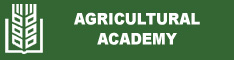GLOBODERA ROSTOCHIENSIS POPULATION DENSITY EFFECT ON POTATO GROWTH AND YIELD. REGRESSION MODELS ESTIMATION
S. Maneva, Zl. Trifonova
Abstract: Effect of initial population densities (Pi) of Bulgarian population of Globodera rostochiensis on the population dynamics and potato plants growth and yield was investigated in glasshouse and estimated using mathematical models. Potato cv. Nadejda seedlings were inoculated with Pi levels of 0, 0.1, 0.2, 0.5, 1, 2, 4, 8, 16, 32, 64 or 128 second stage juveniles/cm3 soil. The relation between Pi and final population density (Pf) was linear and DWLS regression described the relation of nematode reproductive index (Ri) and Pi. The linear regression with lg(X+I) transformation has been used to model the Pi and Pf effect on both, potato height and yield. Tolerance limit of potato to G. rostochiensis was between 0.5 and 1 juveniles/cm3soil. The reduction of yield was significant different at 1.0 Pi. The model predicted that 4.0 Pi caused 50% yield loss and the maximal yield suppression of 87.6% occurred at 128 Pi. Maximum Ri of 27.0 occurred at 4.0 Pi, suggesting an interspecies competition.
Keywords: G. rostochiensis; mathematical model; population density; potato growth; yield loss
Date published: 2017-09-18